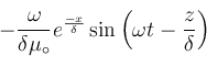Courses

# Maxwell's Equation MCQ Level - 2

## 10 Questions MCQ Test Topic wise Tests for IIT JAM Physics | Maxwell's Equation MCQ Level - 2

Description
This mock test of Maxwell's Equation MCQ Level - 2 for Physics helps you for every Physics entrance exam. This contains 10 Multiple Choice Questions for Physics Maxwell's Equation MCQ Level - 2 (mcq) to study with solutions a complete question bank. The solved questions answers in this Maxwell's Equation MCQ Level - 2 quiz give you a good mix of easy questions and tough questions. Physics students definitely take this Maxwell's Equation MCQ Level - 2 exercise for a better result in the exam. You can find other Maxwell's Equation MCQ Level - 2 extra questions, long questions & short questions for Physics on EduRev as well by searching above.
QUESTION: 1

### A parallel plate capacitor is being discharged. What is the direction of the energy flow in terms of the Poynting vector in the space between the plates?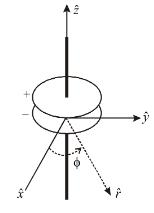Solution: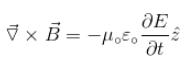As the capacitor is being discharged, field is decreasing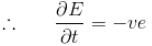Using expression for curl in cylindrical coordinates, we get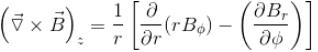Since there is φ symmetry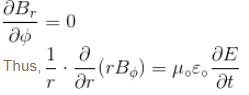Thus, magnetic field in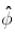direction will be induced, since,
∴ Direction of poynting vector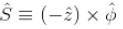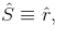radially outward

The correct answer is: Radially outward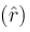QUESTION: 2

### Magnetic field in a region is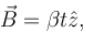the induced electric field due to this time varying magnetic field is

Solution:

The induced electric field lines are concentric circles with clockwise direction.
Physical argument for the direction is that if we put a conducting loop in the magnetic field current will flow in clockwise direction.
∴  Along a circle of radius r, we get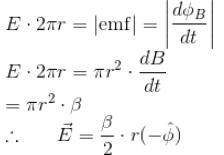Here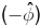means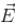is in each a direction to oppose change in flux.
The correct answer is: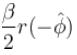QUESTION: 3

### Consider a capacitor placed in free space, consisting of two concentric circular parallel plate of radii r. The separation d  between the plates. The capacitor is connected to an oscillating voltage source of voltage  v(t) = V0 sin(ωt). The magnetic field vector between the plate at a distance r/2 from the axis of the capacitor.

Solution: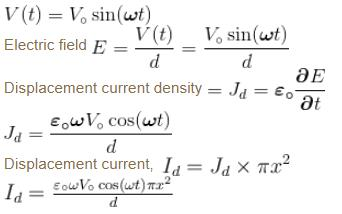Let us take a loop of radius x with centre on the axis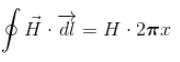Current enclosed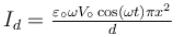Applying Maxwell’s equation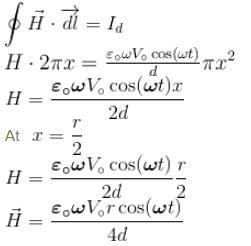The correct answer is: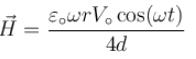QUESTION: 4

Consider the following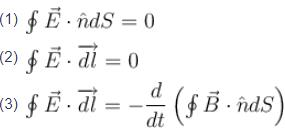where E = electric field and B = magnetic field. Which of the above equations predict(s) the electric field E to be zero?

Solution: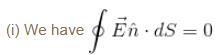Electric flux is zero. It may happen for E ⊥ ds.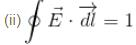Potential difference is zero.
E is not necessarily zero.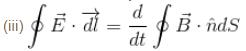⇒ E is produced, if magnetic flux changes with time. Thus, no equation gives E as zero.

The correct answer is: None of these

QUESTION: 5

The magnetic field associated with the electric field vector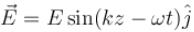is given by

Solution: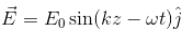Let us use the equation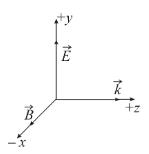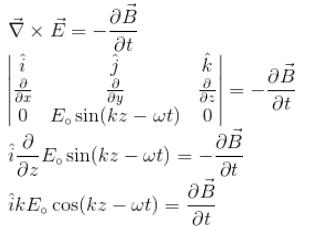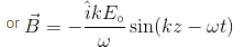(After integration)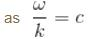The correct answer is: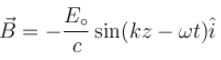QUESTION: 6

A long straight solenoid has n turns per unit length. An alternating current I = Imsinωt flows through it. Find the displacement current density as a function of distance r from the solenoid axis. The cross-sectional radius of solenoid equals R

Solution:

Magnetic field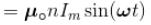To find electric field inside the solenoid, consider a closed loop C, of radius r.
Flux of magnetic field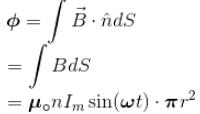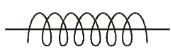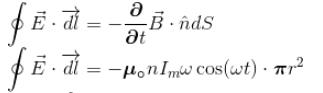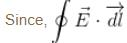is negative, so,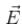lies opposite to the orientation.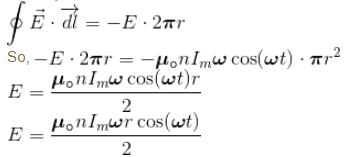Displacement current density,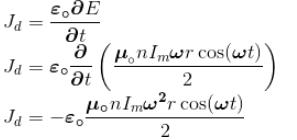Displacement current through a circle of radius r.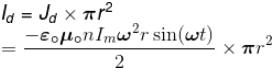The correct answer is: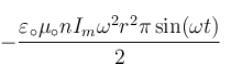QUESTION: 7

Which of the following four equations show that (i) the magnetic field lines cannot start from a point nor end at a point and (ii) the electrostatic field lines cannot form closed loops?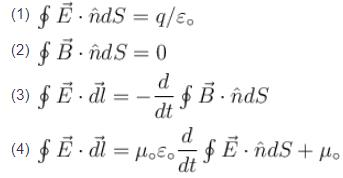Select the correct answer using the codes given below :

Solution:

The magnetic field lines cannot start from a point nor end at a point, i,e., there are no magnetic mono poles. Magnetic filed lines have no source or sink to start or stop.
Thus,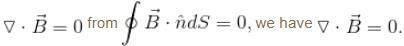If electric field lines cannot form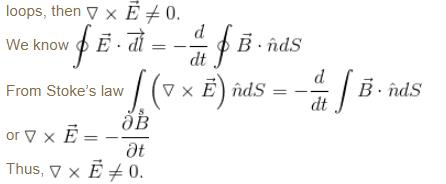The correct answer is: 2 and 3, respectively

QUESTION: 8

An electromagnetic wave travels along z-axis. Which of the following pair of space and time varying fields would generate such a wave?

Solution: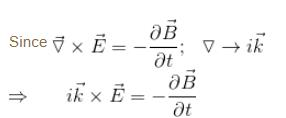As direction of propagation is along z-axis.
E must be in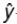-direction, so that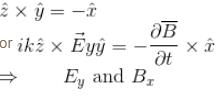QUESTION: 9

LASER are light source which give almost perfectly parallel beam of high intensity. If a 2kW laser beam is concentrated by a lens into cross-sectional area about 10–6 cm2, then the value of Poynting vector

Solution:

Power = 2 kW = 2 × 103 W
Cross-sectional area = 106 cm2
Poynting vector S = P/A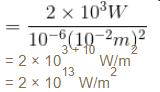The correct answer is: 2 × 1013 W/m2

QUESTION: 10

A plane electromagnetic wave in free space is specified by the electric field.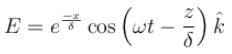The associated magnetic field is

Solution:

Electric field is given by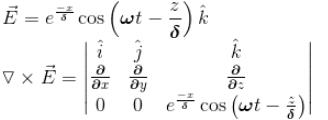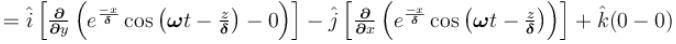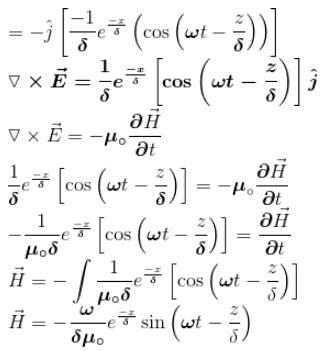The correct answer is: